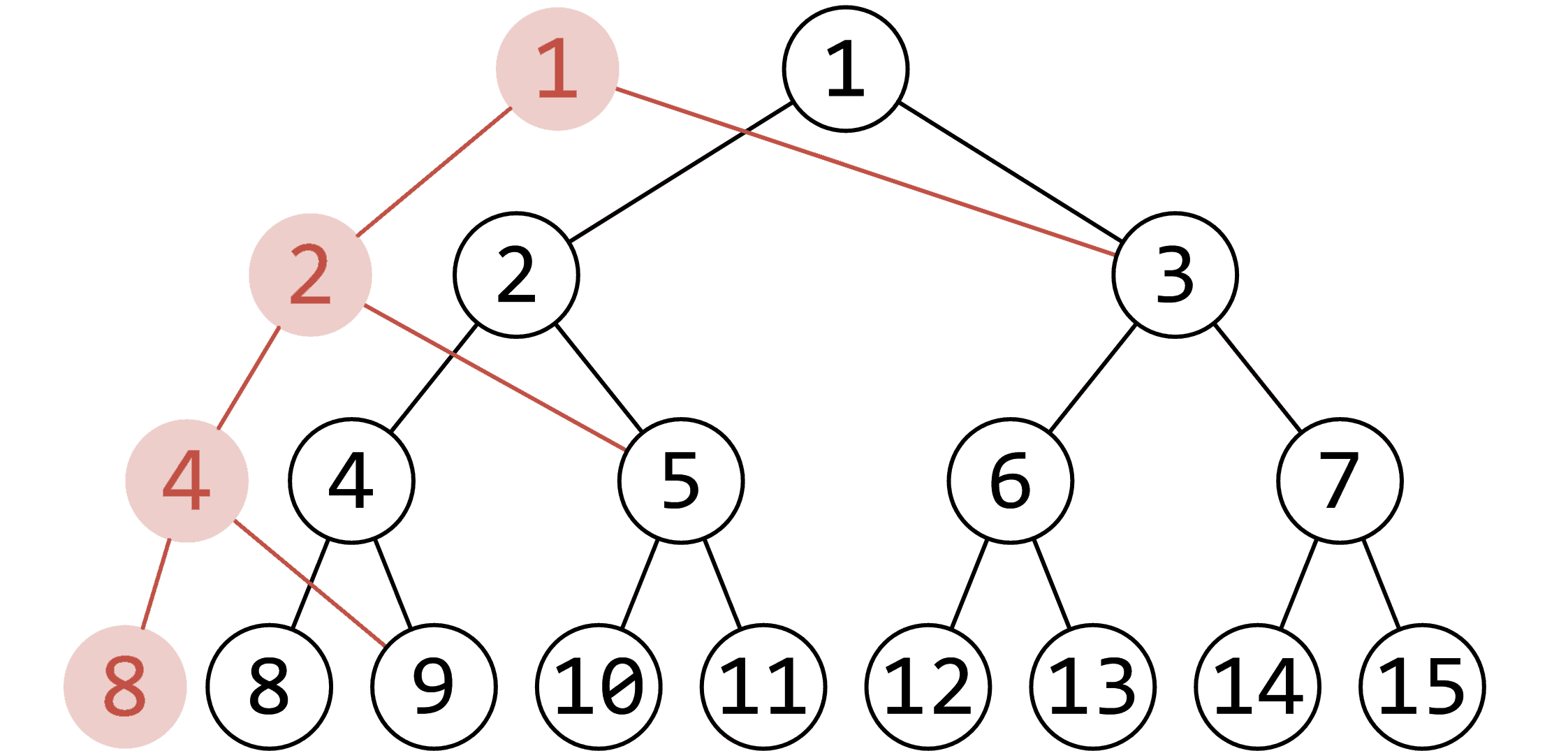# 可持久化线段树

## 主席树¶

（例如下图，修改了 中对应权值为 1 的结点，红色的点即为更改的点）1 2 3 4 5 6 7 8 9 10 11 12 13 14 15 16 17 18 19 20 21 22 23 24 25 26 27 28 29 30 31 32 33 34 35 36 37 38 39 40 41 42 43 44 45 46 47 48 49 50 51 52 53 54 55 56 57 58 59 60 61 62 63 64 65 66 67 68 69 #include #include #include using namespace std; const int maxn = 1e5; // 数据范围 int tot, n, m; int sum[(maxn << 5) + 10], rt[maxn + 10], ls[(maxn << 5) + 10], rs[(maxn << 5) + 10]; int a[maxn + 10], ind[maxn + 10], len; inline int getid(const int &val) { // 离散化 return lower_bound(ind + 1, ind + len + 1, val) - ind; } int build(int l, int r) { // 建树 int root = ++tot; if (l == r) return root; int mid = l + r >> 1; ls[root] = build(l, mid); rs[root] = build(mid + 1, r); return root; // 返回该子树的根节点 } int update(int k, int l, int r, int root) { // 插入操作 int dir = ++tot; ls[dir] = ls[root], rs[dir] = rs[root], sum[dir] = sum[root] + 1; if (l == r) return dir; int mid = l + r >> 1; if (k <= mid) ls[dir] = update(k, l, mid, ls[dir]); else rs[dir] = update(k, mid + 1, r, rs[dir]); return dir; } int query(int u, int v, int l, int r, int k) { // 查询操作 int mid = l + r >> 1, x = sum[ls[v]] - sum[ls[u]]; // 通过区间减法得到左儿子中所存储的数值个数 if (l == r) return l; if (k <= x) // 若 k 小于等于 x ，则说明第 k 小的数字存储在在左儿子中 return query(ls[u], ls[v], l, mid, k); else // 否则说明在右儿子中 return query(rs[u], rs[v], mid + 1, r, k - x); } inline void init() { scanf("%d%d", &n, &m); for (int i = 1; i <= n; ++i) scanf("%d", a + i); memcpy(ind, a, sizeof ind); sort(ind + 1, ind + n + 1); len = unique(ind + 1, ind + n + 1) - ind - 1; rt = build(1, len); for (int i = 1; i <= n; ++i) rt[i] = update(getid(a[i]), 1, len, rt[i - 1]); } int l, r, k; inline void work() { while (m--) { scanf("%d%d%d", &l, &r, &k); printf("%d\n", ind[query(rt[l - 1], rt[r], 1, len, k)]); // 回答询问 } } int main() { init(); work(); return 0; } 

## 拓展：基于主席树的可持久化并查集¶

  1 2 3 4 5 6 7 8 9 10 11 12 13 14 15 16 17 18 19 20 21 22 23 24 25 26 27 28 29 30 31 32 33 34 35 36 37 38 39 40 41 42 43 44 45 46 47 48 49 50 51 52 53 54 55 56 57 58 59 60 61 62 63 64 65 66 67 68 69 70 71 72 73 74 75 76 77 78 79 80 81 82 83 #include #include const int N = 1e5, M = 2e5; // 数据范围 struct node { int val, rank, lchild, rchild; } tree[4 * N + M * 19]; // 每次操作增加节点数最多为 log2(4 * N) = 19 int init[N + 1], root[M + 1], tot; int build(int l, int r) { // 建树 int now = ++tot; if (l == r) { tree[now].val = init[l]; tree[now].rank = 1; return now; } int mid = l + r >> 1; tree[now].lchild = build(l, mid); tree[now].rchild = build(mid + 1, r); return now; } int update(int x, int l, int r, int target, int newroot) { // 合并操作-更新父亲节点信息 int now = ++tot; tree[now] = tree[x]; if (l == r) { tree[now].val = newroot; tree[newroot].rank = std::max(tree[newroot].rank, tree[now].rank + 1); return now; } int mid = l + r >> 1; if (target <= mid) tree[now].lchild = update(tree[x].lchild, l, mid, target, newroot); else tree[now].rchild = update(tree[x].rchild, mid + 1, r, target, newroot); return now; } int query(int x, int l, int r, int target) { // 查询父亲节点 if (l == r) return tree[x].val; int mid = l + r >> 1; if (target <= mid) return query(tree[x].lchild, l, mid, target); else return query(tree[x].rchild, mid + 1, r, target); } int getroot(int x, int l, int r, int target) { // 查询根节点 int ans = query(x, l, r, target); if (ans == target) return ans; else return getroot(x, l, r, ans); } int main() { int n, m; scanf("%d%d", &n, &m); for (int i = 1; i <= n; i++) init[i] = i; root = build(1, n); int op, a, b, k; for (int i = 1; i <= m; i++) { scanf("%d", &op); if (op == 1) { scanf("%d%d", &a, &b); int root_a = getroot(root[i - 1], 1, n, a); int root_b = getroot(root[i - 1], 1, n, b); if (tree[root_a].rank < tree[root_b].rank) // 按秩合并 root[i] = update(root[i - 1], 1, n, root_a, root_b); else root[i] = update(root[i - 1], 1, n, root_b, root_a); } else if (op == 2) { scanf("%d", &k); root[i] = root[k]; } else { scanf("%d%d", &a, &b); root[i] = root[i - 1]; printf("%d\n", getroot(root[i], 1, n, a) == getroot(root[i], 1, n, b)); } } } 

## 参考¶

https://en.wikipedia.org/wiki/Persistent_data_structure

https://www.cnblogs.com/zinthos/p/3899565.html